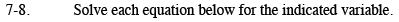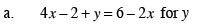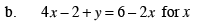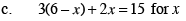### Home > CAAC > Chapter 7 > Lesson 7.1.1 > Problem7-8

7-8.Add 2 to both sides.
4x + y = 8 − 2x

Subtract 4x from both sides.

y = 8 − 6xFollow the steps in part (a).

$x = -\frac{1}{6}y+\frac{8}{6}$Multiply to remove the parentheses then follow the steps in part (a).